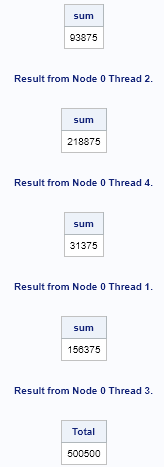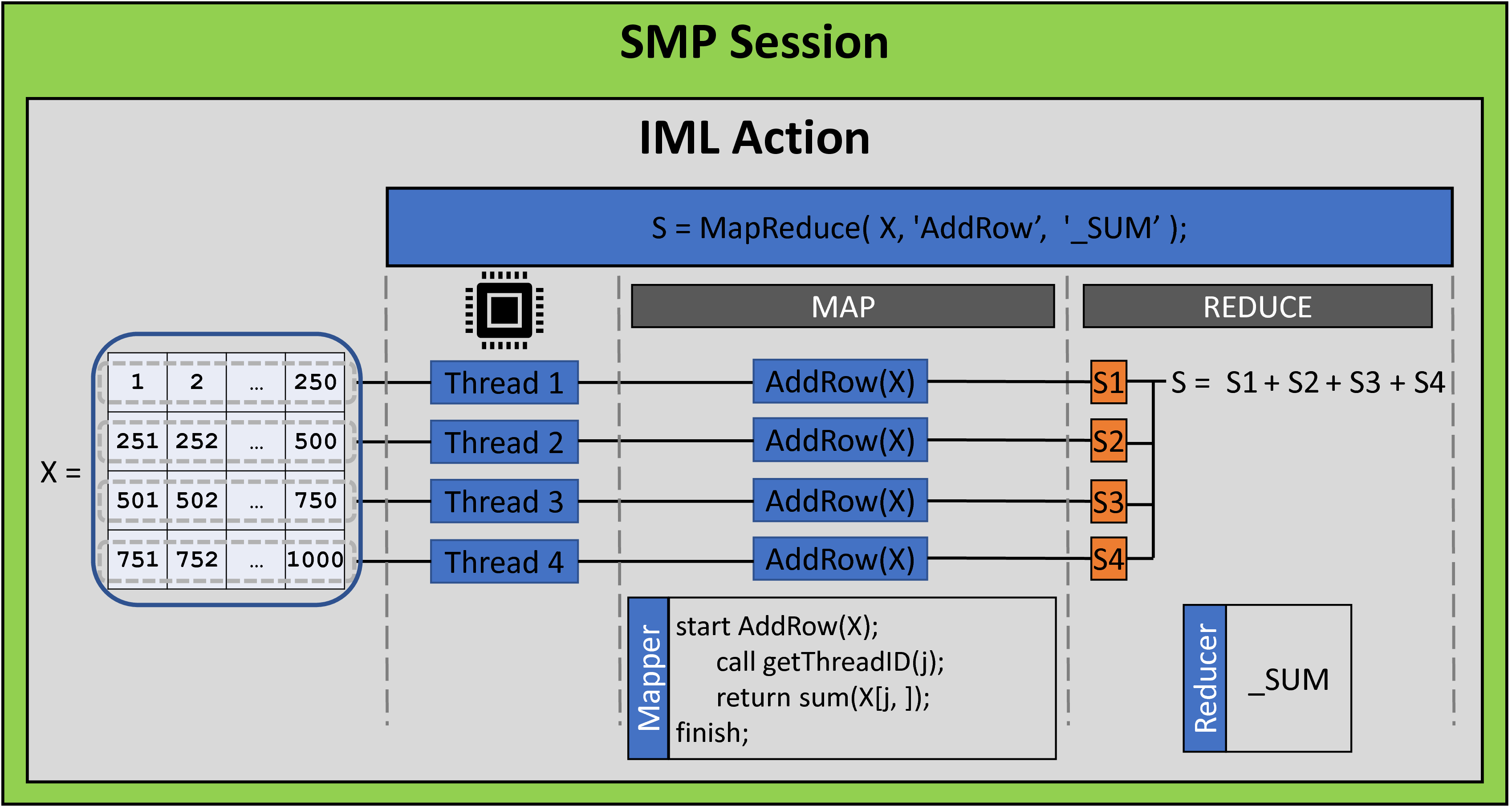The iml action in SAS Viya (introduced in Viya 3.5) provides a set of general programming tools that you can use to implement a custom parallel algorithm. This makes the iml action different than other Viya actions, which use distributed computations to solve specific problems in statistics, machine learning, and artificial intelligence. By using the iml action, you can use programming statements to define the problem you want to solve. One of the simplest ways to run a parallel program is to use the MAPREDUCE function in the iml action, which enables you to distribute a computation across threads and nodes. This article describes the MAPREDUCE function and gives an example.

### What is the map-reduce paradigm?

The MAPREDUCE function implements the map-reduce paradigm, which is a two-step process for distributing a computation. The MAPREDUCE function runs a SAS/IML module (called the mapping function, or the mapper) on every available node and thread in your CAS session. Each mapping function returns a result. The results are aggregated by using a reducing function (the reducer). The final aggregated result is returned by the MAPREDUCE function. The MAPREDUCE function is ideal for “embarrassingly parallel” computations, which are composed of many independent and essentially identical computations. Examples in statistics include Monte Carlo simulation and resampling methods such as the bootstrap. A Wikipedia article about the map-reduce framework includes other examples and more details.

### A simple map-reduce example: Adding numbers

You can use the MAPREDUCE function in the iml action to implement the map-reduce paradigm. The syntax of the MAPREDUCE function is
result = MAPREDUCE( mapArg, 'MapFunc', 'RedFunc' );
In this syntax, 'MapFunc' is the name of the mapping function and mapArg is a parameter that is passed to the mapper function in every thread. The 'RedFunc' argument is the name of the reducing function. You can use a predefined (built-in) reducers, or you can define your own reducer. This article uses only predefined reducers.

Let's implement the sum-of-N-numbers algorithm in the iml action by using four threads to sum the numbers 1, 2, ..., 1000. (For simplicity, I chose N divisible by 4, so each thread sums N/4 = 250 numbers.) There are many ways to send data to the mapper. This program packs the data into a matrix that has four rows and tells each thread to analyze one row. The program defines a helper function (getThreadID) and a mapper function (AddRow), which will run on each thread. The AddRow function does the following:

1. Call the getThreadID function to find the thread in which the AddRow function is running. The getThreadID function is a thin wrapper around the NODEINFO function, which is a built-in function in the iml action. The thread ID is stored in the variable j.
2. Extract the j th row of numbers. These are the numbers that the thread will sum.
3. Call the SUM function to compute the partial sum. Return that value.

In the following program, the built-in '_SUM' reducer adds the partial sums and returns the total sum. The program prints the result from each thread. Because the computation is performed in parallel, the order of the output is arbitrary and can vary from run to run. If you are not familiar with using PROC CAS to call the iml action, see the getting started example for the iml action.

```/* assume SESS0 is a CAS session that has 0 workers (controller only) and at least 4 threads */ proc cas; session sess0; /* SMP session: controller node only */ loadactionset 'iml'; /* load the action set */ source MapReduceAdd; start getThreadID(j); /* this function runs on the j_th thread */ j = nodeInfo()\$'threadId'; /* put thread ID into the variable j */ finish; start AddRow(X); call getThreadId(j); /* get thread ID */ sum = sum(X[j, ]); /* compute the partial sum for the j_th row */ print sum; /* print partial sum for this thread */ return sum; /* return the partial sum */ finish;   /* ----- Main Program ----- */ x = shape(1:1000, 4); /* create a 4 x 250 matrix */ Total = MapReduce(x, 'AddRow', '_SUM'); /* use built-in _SUM reducer */ print Total; endsource; iml / code=MapReduceAdd nthreads=4; run;```The output shows the results of the PRINT statement in each thread. The output can appear in any order. For this run, the first output is from the second thread, which computes the sum of the numbers 251, 252, . . . , 500 and returns the value 93,875. The next output is from the fourth thread, which computes the sum of the numbers 751, 752, . . . , 1000. The other threads perform similar computations. The partial sums are sent to the built-in '_SUM' reducer, which adds them together and returns the total sum to the main program. The total sum is 500,500 and appears at the end of the output.

### Visualize the map-reduce computations

You can use the following diagram to help visualize the program. (Click to enlarge.)The following list explains parts of the diagram:

• My CAS session did not include any worker nodes. Therefore, the iml action runs entirely on the controller, although it can still use multiple threads. This mode is known as symmetric multiprocessing (SMP) or single-machine mode. Notice that the call to the iml action (the statement just before the RUN statement) specifies the NTHREADS=4 parameter, which causes the action to use four threads.
• The program defines the matrix X, which has four rows. This matrix is sent to each thread.
• Each thread runs the AddRow function (the mapper). The function uses the NODEINFO function to determine the thread it is running in. It then sums the corresponding row of the X matrix and returns that partial sum.
• The reducer combines the results of the four mappers. In this case, the reducer is '_SUM', so the reducer adds the four partial sums. This sum is the value that is returned by the MAPREDUCE function.

### Summary